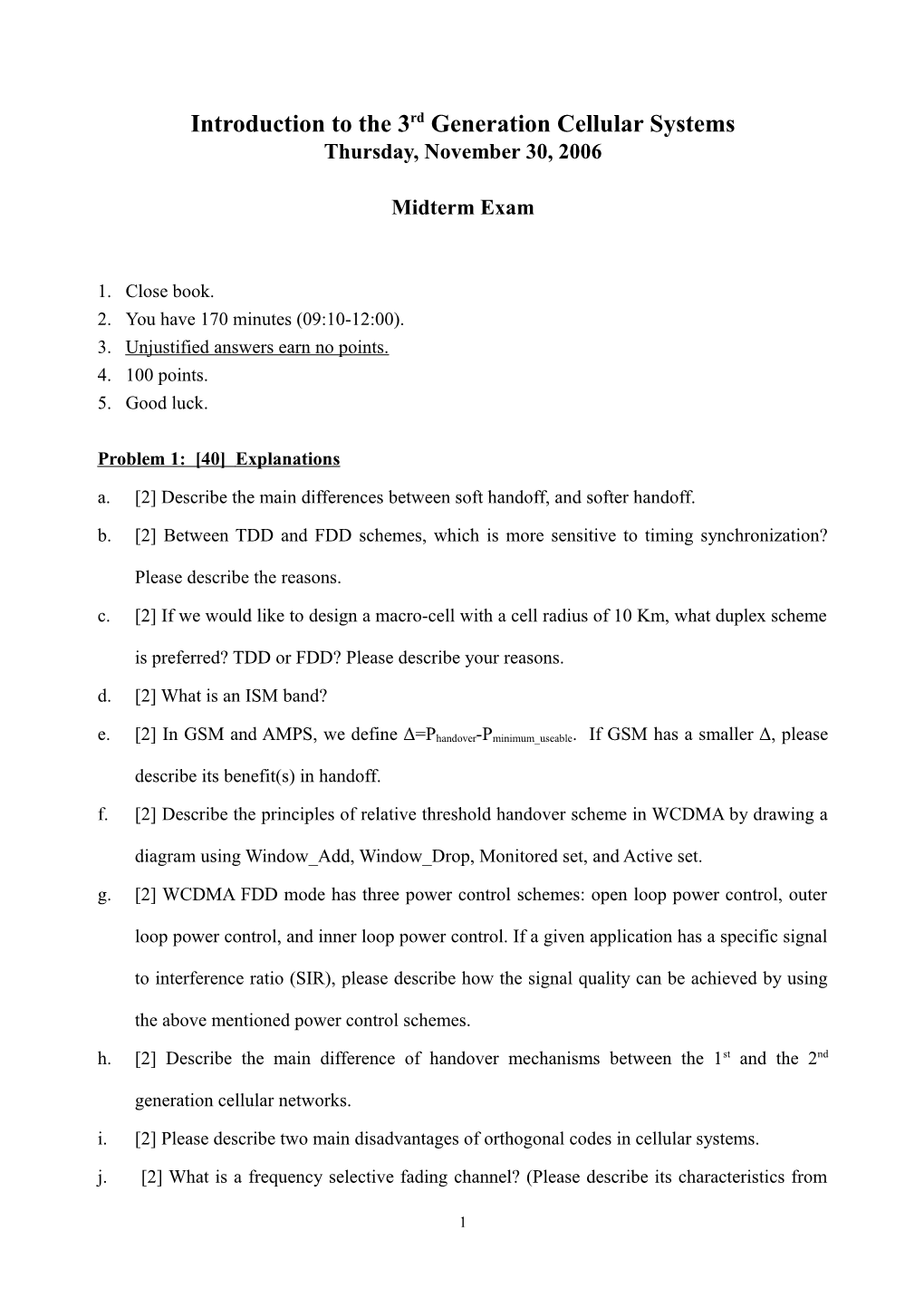# Introduction to the 3Rd Generation Cellular SystemsIntroduction to the 3rd Generation Cellular Systems

Thursday, November 30, 2006

Midterm Exam

1. Close book.
2. You have 170 minutes (09:10-12:00).
3. Unjustified answers earn no points.
4. 100 points.
5. Good luck.

Problem 1:  Explanations

1.  Describe the main differences between soft handoff, and softer handoff.
2.  Between TDD and FDD schemes, which is more sensitive to timing synchronization? Please describe the reasons.
3.  If we would like to design a macro-cell with a cell radius of 10 Km, what duplex scheme is preferred? TDD or FDD? Please describe your reasons.
4.  What is an ISM band?
5.  In GSM and AMPS, we define Δ=Phandover-Pminimum_useable. If GSM has a smaller Δ, please describe its benefit(s) in handoff.
6.  Describe the principles of relative threshold handover scheme in WCDMA by drawing a diagram using Window_Add, Window_Drop, Monitored set, and Active set.
7.  WCDMA FDD mode has three power control schemes: open loop power control, outer loop power control, and inner loop power control. If a given application has a specific signal to interference ratio (SIR), please describe how the signal quality can be achieved by using the above mentioned power control schemes.
8.  Describe the main difference of handover mechanisms between the 1st and the 2nd generation cellular networks.
9.  Please describe two main disadvantages of orthogonal codes in cellular systems.
10.  What is a frequency selective fading channel? (Please describe its characteristics from both time and frequency domain.)
11.  What is a time selective fading channel? (Please describe its characteristics from both time and frequency domain.)
12.  What is a hybrid channel assignment scheme?
13.  Please describe the meaning of processing gain.
14.  What is the definition of root mean squared (RMS) delay spread?
16.  What is the level crossing rate?
17.  Please describe the two open loop transmit diversity schemes in the downlink of WCDMA.
18.  Please describe the near-far effect.
19.  Please describe the Jake’s model by drawing a figure and related parameters.

Problem 2. Rayleigh Distribution

Consider a carrier signal s at a frequency and an amplitude a:

The received signal is the sum of n waves:

(a).  Show that the pdf of a Rayleigh distribution is given by

Please write down all the necessary assumptions in obtaining this result.

(b).  Please derive the mean squared value of r.

Problem 3.  Reuse Ratio

Prove that for a hexagonal geometry, the co-channel reuse ratio is given by .

Problem 4.  Trunking Theory:

A hexagonal cell within a 4-cell system has a radius of 1.387 km. A total of 60 channels are used within the entire system. If the load per user is 0.029 Erlangs, and call/hour, compute the following for an Erlang C system with a GOS of 5%. (An Erlang C chart is given in Page 4.)

(a). How many users per square kilometer will this system support?

(b). What is the probability that a delayed call will have to wait for more than 10 seconds?

Hint:

(c). What is the probability that a call will be delayed for more than 10 seconds?

Problem 5.  Orthogonal Codes and Frequency Selective Fading Channels

As shown in the attached reference, an orthogonal code hopping multiplexing (OCHM) scheme is proposed for the downlink of a direct-sequence code division multiple access (DS-CDMA) system, where simulation experiments are conducted for additive while Gaussian noise (AWGN) channels. However, in systems using CDMA technologies, channels are usually modeled as frequency selective fading. In your opinion, is the proposed system architecture suitable for frequency selective fading channels? Please justify your answers.1